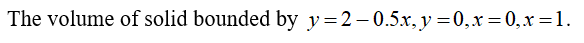# Find the volume V of the solid obtained by rotating the region bounded by the given curves about the specified line.y = 2 − 0.5x, y = 0, x = 0, x = 1;    about the x-axis

Question
1 views

Find the volume V of the solid obtained by rotating the region bounded by the given curves about the specified line.

y = 2 − 0.5x, y = 0, x = 0, x = 1;
check_circle

Given:...

### Want to see the full answer?

See Solution

#### Want to see this answer and more?

Solutions are written by subject experts who are available 24/7. Questions are typically answered within 1 hour.*

See Solution
*Response times may vary by subject and question.
Tagged in

### Calculus